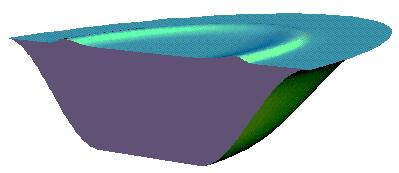Search IntMath
Close

# Modeling tsunamis

By Murray Bourne, 18 Jan 2007

Here's an interesting article on the use of Mathematica to model the wave action of a tsunami.

Such a model involves a system of coupled partial differential equations. The computing power required for such calculations is awesome.You may like to see an introduction to differential equations. That chapter does not get up to partial DEs, nor does it talk about systems of DEs, both of which are fascinating topics. But it does give you an idea of what differential equations are about and what they are used for.

### Comment Preview

HTML: You can use simple tags like <b>, <a href="...">, etc.

To enter math, you can can either:

1. Use simple calculator-like input in the following format (surround your math in backticks, or qq on tablet or phone):
a^2 = sqrt(b^2 + c^2)
(See more on ASCIIMath syntax); or
2. Use simple LaTeX in the following format. Surround your math with $$ and $$.
$$\int g dx = \sqrt{\frac{a}{b}}$$
(This is standard simple LaTeX.)

NOTE: You can mix both types of math entry in your comment.